# 5.2 Unit circle: sine and cosine functions  (Page 8/12)

 Page 8 / 12

$\mathrm{sin}\text{\hspace{0.17em}}\frac{\pi }{2}$

$\mathrm{sin}\text{\hspace{0.17em}}\frac{\pi }{3}$

$\frac{\sqrt{3}}{2}$

$\mathrm{cos}\text{\hspace{0.17em}}\frac{\pi }{2}$

$\mathrm{cos}\text{\hspace{0.17em}}\frac{\pi }{3}$

$\frac{1}{2}$

$\mathrm{sin}\text{\hspace{0.17em}}\frac{\pi }{4}$

$\mathrm{cos}\text{\hspace{0.17em}}\frac{\pi }{4}$

$\frac{\sqrt{2}}{2}$

$\mathrm{sin}\text{\hspace{0.17em}}\frac{\pi }{6}$

$\mathrm{sin}\text{\hspace{0.17em}}\pi$

0

$\mathrm{sin}\text{\hspace{0.17em}}\frac{3\pi }{2}$

$\mathrm{cos}\text{\hspace{0.17em}}\pi$

−1

$\mathrm{cos}\text{\hspace{0.17em}}0$

$\mathrm{cos}\text{\hspace{0.17em}}\frac{\pi }{6}$

$\frac{\sqrt{3}}{2}$

$\mathrm{sin}\text{\hspace{0.17em}}0$

## Numeric

For the following exercises, state the reference angle for the given angle.

$240°$

$60°$

$-170°$

$100°$

$80°$

$-315°$

$135°$

$45°$

$\frac{5\pi }{4}$

$\frac{2\pi }{3}$

$\frac{\pi }{3}$

$\frac{5\pi }{6}$

$\frac{-11\pi }{3}$

$\frac{\pi }{3}$

$\frac{-\text{\hspace{0.17em}}7\pi }{4}$

$\frac{-\pi }{8}$

$\frac{\pi }{8}$

For the following exercises, find the reference angle, the quadrant of the terminal side, and the sine and cosine of each angle. If the angle is not one of the angles on the unit circle, use a calculator and round to three decimal places.

$225°$

$300°$

$60°,$ Quadrant IV, $\text{sin}\left(300°\right)=-\frac{\sqrt{3}}{2},\mathrm{cos}\left(300°\right)=\frac{1}{2}\text{\hspace{0.17em}}$

$320°$

$135°$

$45°,$ Quadrant II, $\text{\hspace{0.17em}}\text{sin}\left(135°\right)=\frac{\sqrt{2}}{2},$ $\text{\hspace{0.17em}}\mathrm{cos}\left(135°\right)=-\frac{\sqrt{2}}{2}$

$210°$

$120°$

$60°,$ Quadrant II, $\text{\hspace{0.17em}}\text{sin}\left(120°\right)=\frac{\sqrt{3}}{2},$ $\text{\hspace{0.17em}}\mathrm{cos}\left(120°\right)=-\frac{1}{2}$

$250°$

$150°$

$\text{\hspace{0.17em}}30°,$ Quadrant II, $\text{\hspace{0.17em}}\text{sin}\left(150°\right)=\frac{1}{2},$ $\text{\hspace{0.17em}}\mathrm{cos}\left(150°\right)=-\frac{\sqrt{3}}{2}$

$\frac{5\pi }{4}$

$\frac{7\pi }{6}$

$\frac{\pi }{6},$ Quadrant III, $\text{\hspace{0.17em}}\text{sin}\left(\frac{7\pi }{6}\right)=-\frac{1}{2},$ $\text{cos}\left(\frac{7\pi }{6}\right)=-\frac{\sqrt{3}}{2}$

$\frac{5\pi }{3}$

$\frac{3\pi }{4}$

$\frac{\pi }{4},$ Quadrant II, $\text{\hspace{0.17em}}\text{sin}\left(\frac{3\pi }{4}\right)=\frac{\sqrt{2}}{2},$ $\text{\hspace{0.17em}}\mathrm{cos}\left(\frac{4\pi }{3}\right)=-\frac{\sqrt[]{2}}{2}$

$\frac{4\pi }{3}$

$\frac{2\pi }{3}$

$\frac{\pi }{3},$ Quadrant II, $\text{\hspace{0.17em}}\text{sin}\left(\frac{2\pi }{3}\right)=\frac{\sqrt{3}}{2},$ $\text{\hspace{0.17em}}\mathrm{cos}\left(\frac{2\pi }{3}\right)=-\frac{1}{2}$

$\frac{5\pi }{6}$

$\frac{7\pi }{4}$

$\frac{\pi }{4},$ Quadrant IV, $\text{\hspace{0.17em}}\text{sin}\left(\frac{7\pi }{4}\right)=-\frac{\sqrt{2}}{2},$ $\text{cos}\left(\frac{7\pi }{4}\right)=\frac{\sqrt{2}}{2}$

For the following exercises, find the requested value.

If $\text{\hspace{0.17em}}\text{cos}\left(t\right)=\frac{1}{7}\text{\hspace{0.17em}}$ and $\text{\hspace{0.17em}}t\text{\hspace{0.17em}}$ is in the 4 th quadrant, find $\text{\hspace{0.17em}}\text{sin}\left(t\right).$

If $\text{\hspace{0.17em}}\text{cos}\left(t\right)=\frac{2}{9}\text{\hspace{0.17em}}$ and $\text{\hspace{0.17em}}t\text{\hspace{0.17em}}$ is in the 1 st quadrant, find $\text{\hspace{0.17em}}\text{sin}\left(t\right).\text{\hspace{0.17em}}$

$\frac{\sqrt{77}}{9}$

If $\text{\hspace{0.17em}}\text{sin}\left(t\right)=\frac{3}{8}\text{\hspace{0.17em}}$ and $\text{\hspace{0.17em}}t\text{\hspace{0.17em}}$ is in the 2 nd quadrant, find $\text{\hspace{0.17em}}\text{cos}\left(t\right).$

If $\text{\hspace{0.17em}}\text{sin}\left(t\right)=-\frac{1}{4}\text{\hspace{0.17em}}$ and $\text{\hspace{0.17em}}t\text{\hspace{0.17em}}$ is in the 3 rd quadrant, find $\text{\hspace{0.17em}}\text{cos}\left(t\right).$

$-\frac{\sqrt{15}}{4}$

Find the coordinates of the point on a circle with radius 15 corresponding to an angle of $\text{\hspace{0.17em}}220°.$

Find the coordinates of the point on a circle with radius 20 corresponding to an angle of $\text{\hspace{0.17em}}120°.$

$\text{\hspace{0.17em}}\left(-10,10\sqrt{3}\right)\text{\hspace{0.17em}}$

Find the coordinates of the point on a circle with radius 8 corresponding to an angle of $\text{\hspace{0.17em}}\frac{7\pi }{4}.$

Find the coordinates of the point on a circle with radius 16 corresponding to an angle of $\text{\hspace{0.17em}}\frac{5\pi }{9}.$

$\text{\hspace{0.17em}}\left(–2.778,15.757\right)\text{\hspace{0.17em}}$

State the domain of the sine and cosine functions.

State the range of the sine and cosine functions.

$\text{\hspace{0.17em}}\text{\hspace{0.17em}}\left[–1,1\right]\text{\hspace{0.17em}}$

## Graphical

For the following exercises, use the given point on the unit circle to find the value of the sine and cosine of $\text{\hspace{0.17em}}t\text{\hspace{0.17em}.}$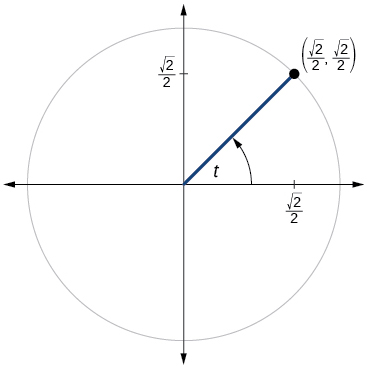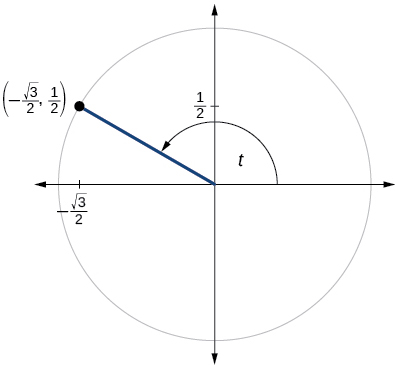$\mathrm{sin}t=\frac{1}{2},\mathrm{cos}t=-\frac{\sqrt{3}}{2}$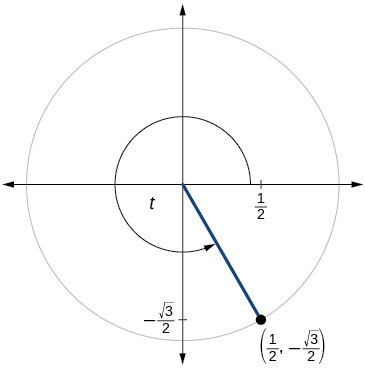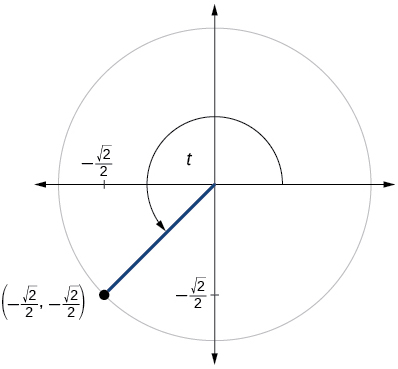$\mathrm{sin}\text{\hspace{0.17em}}t=-\frac{\sqrt{2}}{2},\mathrm{cos}\text{\hspace{0.17em}}t=-\frac{\sqrt{2}}{2}$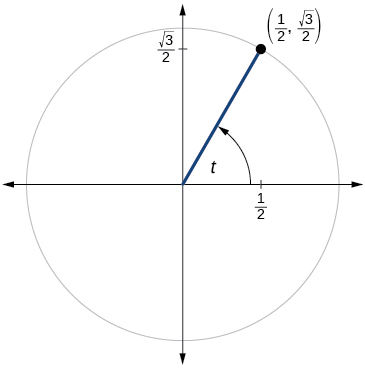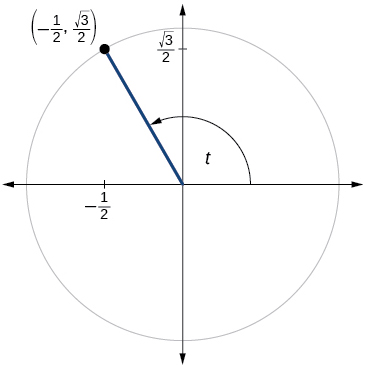$\mathrm{sin}\text{\hspace{0.17em}}t=\frac{\sqrt{3}}{2},\mathrm{cos}\text{\hspace{0.17em}}t=-\frac{1}{2}$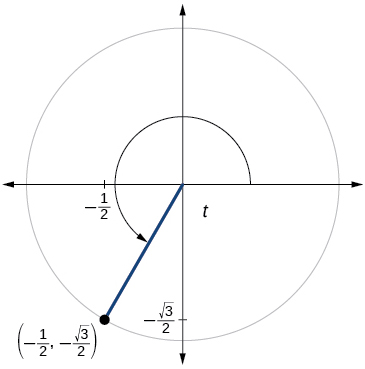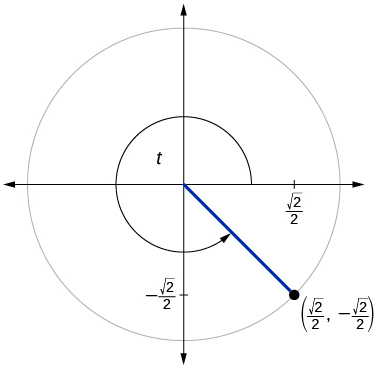$\mathrm{sin}\text{\hspace{0.17em}}t=-\frac{\sqrt{2}}{2},\mathrm{cos}\text{\hspace{0.17em}}t=\frac{\sqrt{2}}{2}$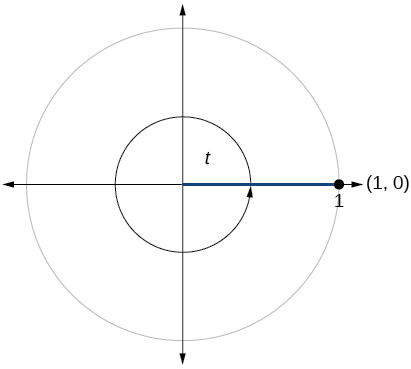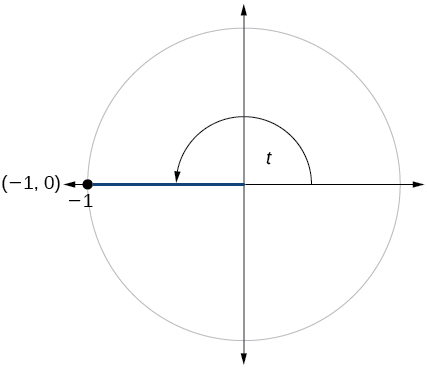$\mathrm{sin}\text{\hspace{0.17em}}t=0,\mathrm{cos}\text{\hspace{0.17em}}t=-1$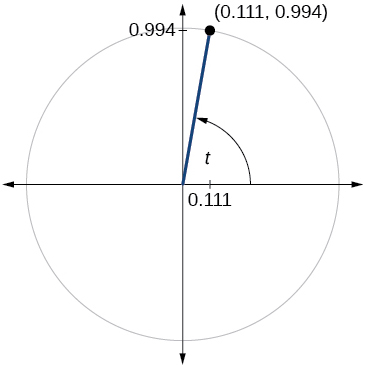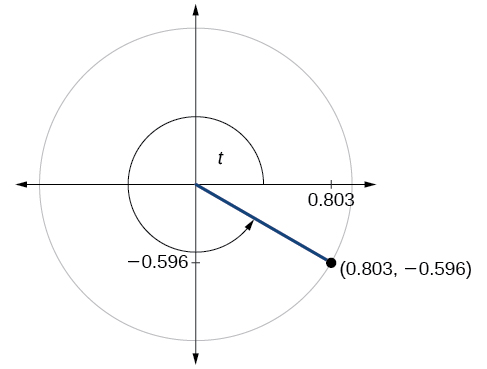$\mathrm{sin}\text{\hspace{0.17em}}t=-0.596,\mathrm{cos}\text{\hspace{0.17em}}t=0.803$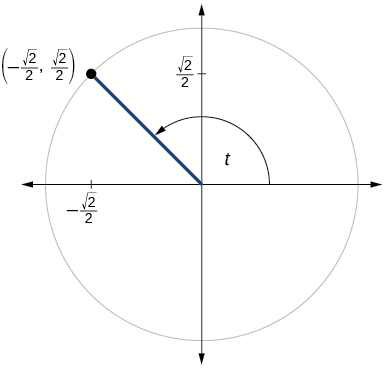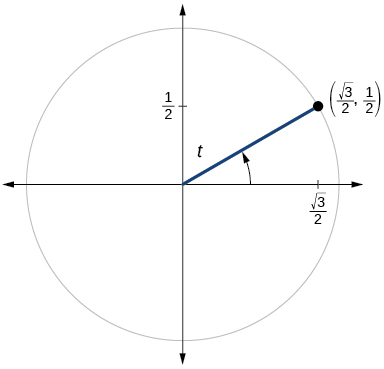$\mathrm{sin}\text{\hspace{0.17em}}t=\frac{1}{2},\mathrm{cos}\text{\hspace{0.17em}}t=\frac{\sqrt{3}}{2}$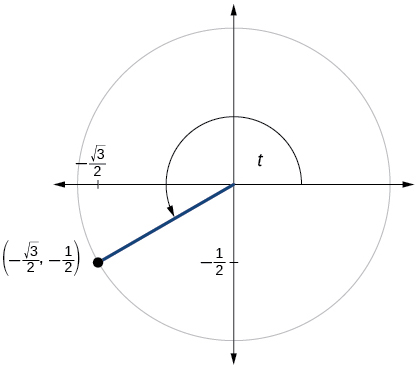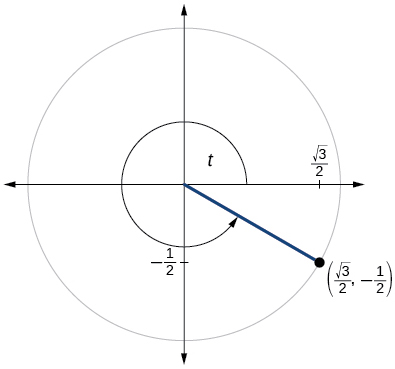$\mathrm{sin}\text{\hspace{0.17em}}t=-\frac{1}{2},\mathrm{cos}\text{\hspace{0.17em}}t=\frac{\sqrt{3}}{2}$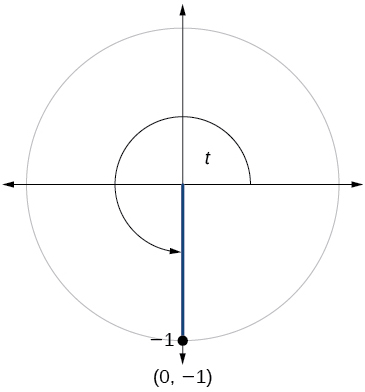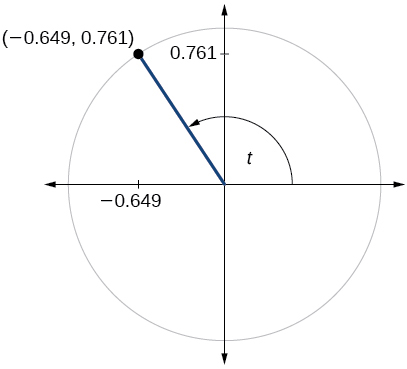$\mathrm{sin}\text{\hspace{0.17em}}t=0.761,\mathrm{cos}\text{\hspace{0.17em}}t=-0.649$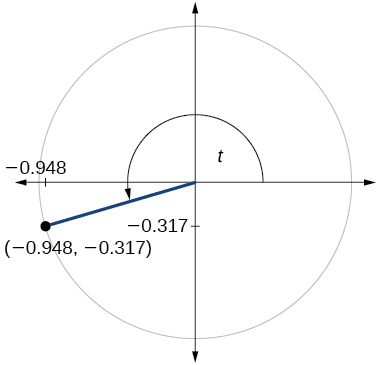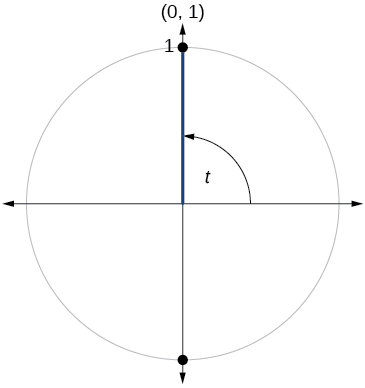$\mathrm{sin}\text{\hspace{0.17em}}t=1,\mathrm{cos}\text{\hspace{0.17em}}t=0$

## Technology

For the following exercises, use a graphing calculator to evaluate.

$\mathrm{sin}\text{\hspace{0.17em}}\frac{5\pi }{9}$

$\mathrm{cos}\text{\hspace{0.17em}}\frac{5\pi }{9}$

−0.1736

$\mathrm{sin}\text{\hspace{0.17em}}\frac{\pi }{10}$

$\mathrm{cos}\text{\hspace{0.17em}}\frac{\pi }{10}$

0.9511

$\mathrm{sin}\text{\hspace{0.17em}}\frac{3\pi }{4}$

$\mathrm{cos}\text{\hspace{0.17em}}\frac{3\pi }{4}$

−0.7071

$\mathrm{sin}\text{\hspace{0.17em}}98°$

$\mathrm{cos}\text{\hspace{0.17em}}98°$

−0.1392

$\mathrm{cos}\text{\hspace{0.17em}}310°$

$\mathrm{sin}\text{\hspace{0.17em}}310°$

−0.7660

## Extensions

$\mathrm{sin}\left(\frac{11\pi }{3}\right)\mathrm{cos}\left(\frac{-5\pi }{6}\right)$

$\mathrm{sin}\left(\frac{3\pi }{4}\right)\mathrm{cos}\left(\frac{5\pi }{3}\right)$

$\frac{\sqrt{2}}{4}$

$\mathrm{sin}\left(-\frac{4\pi }{3}\right)\mathrm{cos}\left(\frac{\pi }{2}\right)$

$\mathrm{sin}\left(\frac{-9\pi }{4}\right)\mathrm{cos}\left(\frac{-\pi }{6}\right)$

$-\frac{\sqrt{6}}{4}$

$\mathrm{sin}\left(\frac{\pi }{6}\right)\mathrm{cos}\left(\frac{-\pi }{3}\right)$

$\mathrm{sin}\left(\frac{7\pi }{4}\right)\mathrm{cos}\left(\frac{-2\pi }{3}\right)$

$\frac{\sqrt{2}}{4}$

$\mathrm{cos}\left(\frac{5\pi }{6}\right)\mathrm{cos}\left(\frac{2\pi }{3}\right)$

$\mathrm{cos}\left(\frac{-\pi }{3}\right)\mathrm{cos}\left(\frac{\pi }{4}\right)$

$\frac{\sqrt{2}}{4}$

$\mathrm{sin}\left(\frac{-5\pi }{4}\right)\mathrm{sin}\left(\frac{11\pi }{6}\right)$

$\mathrm{sin}\left(\pi \right)\mathrm{sin}\left(\frac{\pi }{6}\right)$

0

## Real-world applications

For the following exercises, use this scenario: A child enters a carousel that takes one minute to revolve once around. The child enters at the point $\text{\hspace{0.17em}}\left(0,1\right),$ that is, on the due north position. Assume the carousel revolves counter clockwise.

What are the coordinates of the child after 45 seconds?

What are the coordinates of the child after 90 seconds?

$\left(0,–1\right)$

What is the coordinates of the child after 125 seconds?

When will the child have coordinates $\text{\hspace{0.17em}}\left(0.707,–0.707\right)\text{\hspace{0.17em}}$ if the ride lasts 6 minutes? (There are multiple answers.)

37.5 seconds, 97.5 seconds, 157.5 seconds, 217.5 seconds, 277.5 seconds, 337.5 seconds

When will the child have coordinates $\text{\hspace{0.17em}}\left(-0.866,-0.5\right)\text{\hspace{0.17em}}$ if the ride last 6 minutes?

what is a function?
I want to learn about the law of exponent
explain this
what is functions?
A mathematical relation such that every input has only one out.
Spiro
yes..it is a relationo of orders pairs of sets one or more input that leads to a exactly one output.
Mubita
Is a rule that assigns to each element X in a set A exactly one element, called F(x), in a set B.
RichieRich
If the plane intersects the cone (either above or below) horizontally, what figure will be created?
can you not take the square root of a negative number
No because a negative times a negative is a positive. No matter what you do you can never multiply the same number by itself and end with a negative
lurverkitten
Actually you can. you get what's called an Imaginary number denoted by i which is represented on the complex plane. The reply above would be correct if we were still confined to the "real" number line.
Liam
Suppose P= {-3,1,3} Q={-3,-2-1} and R= {-2,2,3}.what is the intersection
can I get some pretty basic questions
In what way does set notation relate to function notation
Ama
is precalculus needed to take caculus
It depends on what you already know. Just test yourself with some precalculus questions. If you find them easy, you're good to go.
Spiro
the solution doesn't seem right for this problem
what is the domain of f(x)=x-4/x^2-2x-15 then
x is different from -5&3
Seid
All real x except 5 and - 3
Spiro
***youtu.be/ESxOXfh2Poc
Loree
how to prroved cos⁴x-sin⁴x= cos²x-sin²x are equal
Don't think that you can.
Elliott
By using some imaginary no.
Tanmay
how do you provided cos⁴x-sin⁴x = cos²x-sin²x are equal
What are the question marks for?
Elliott
Someone should please solve it for me Add 2over ×+3 +y-4 over 5 simplify (×+a)with square root of two -×root 2 all over a multiply 1over ×-y{(×-y)(×+y)} over ×y
For the first question, I got (3y-2)/15 Second one, I got Root 2 Third one, I got 1/(y to the fourth power) I dont if it's right cause I can barely understand the question.
Is under distribute property, inverse function, algebra and addition and multiplication function; so is a combined question
Abena
find the equation of the line if m=3, and b=-2
graph the following linear equation using intercepts method. 2x+y=4
Ashley
how
Wargod
what?
John
ok, one moment
UriEl
how do I post your graph for you?
UriEl
it won't let me send an image?
UriEl
also for the first one... y=mx+b so.... y=3x-2
UriEl
y=mx+b you were already given the 'm' and 'b'. so.. y=3x-2
Tommy
Please were did you get y=mx+b from
Abena
y=mx+b is the formula of a straight line. where m = the slope & b = where the line crosses the y-axis. In this case, being that the "m" and "b", are given, all you have to do is plug them into the formula to complete the equation.
Tommy
thanks Tommy
Nimo
0=3x-2 2=3x x=3/2 then . y=3/2X-2 I think
Given
co ordinates for x x=0,(-2,0) x=1,(1,1) x=2,(2,4)
neilByByBy OpenStaxBy OpenStaxBy Anh DaoBy Jonathan LongBy OpenStaxByBy Carly AllenBy Rohini AjayBy Hope PercleBy OpenStax